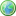Accessibility options:

# Differential Equations resources

Show me all resources applicable to

### Facts & Formulae Leaflets (1)Mathematical Tools for Physical Sciences and Systems Biology
This leaflet is a summary of common mathematical definitions and properties used in the Physical Sciences and Systems Biology contributed to the mathcentre Community Project by Dr Morgiane Richard, University of Aberdeen and reviewed by Mamen Romano and Ian Stansfield, University of Aberdeen.

### Teach Yourself (1)Second Order Ordinary Differential Equations
Second Order Differential Equations: A summary of how to solve second order ODEs with constant coefficients: how to solve homogeneous equations (with no right hand-side) and how to solve equations with a right hand-side (method of undetermined coefficients and methods of variation of parameters). This Teach Yourself resource is contributed to the mathcentre Community Project by Morgiane Richard, University of Aberdeen and is reviewed by Shazia Ahmed, University of Glasgow.

### Test Yourself (3)First order differential equations - Numbas
9 questions on first order differential equations. Straight forward integration (2), separating variables (4), linear (1), homogenous (2). All are either initial value or boundary value problems. Numbas resources have been made available under a Creative Commons licence by Bill Foster and Christian Perfect, School of Mathematics & Statistics at Newcastle University.First order differential equations - Numbas
9 questions on first order differential equations. Straight forward integration (2), separating variables (4), linear (1), homogenous (2). All are either initial value or boundary value problems. Numbas resources have been made available under a Creative Commons licence by the School of Mathematics & Statistics at Newcastle University.Second order differential equations - Numbas
5 questions on second order differential equations. First two and last, linear with constant coefficients; first two homogeneous, complex and repeated roots. Last, non-homogeneous. Third, motion under gravity. Fourth, linear with a given particular solution (variation of parameters). Numbas resources have been made available under a Creative Commons licence by the School of Mathematics & Statistics at Newcastle University.

Website design by Pink Mayhem, Leicester